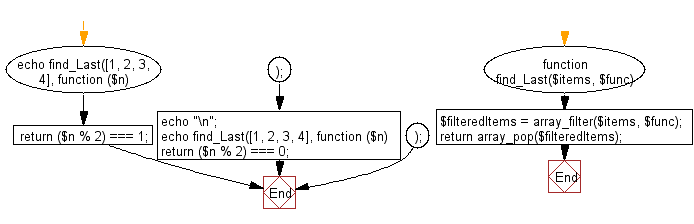﻿ PHP Exercise: Get the last element for which the given function returns a truth value - w3resource

# PHP Exercises: Get the last element for which the given function returns a truth value

## PHP: Exercise-81 with Solution

Write a PHP program to get the last element for which the given function returns a truth value.

Sample Solution: -

PHP Code:

``````<?php
function find_Last(\$items, \$func)
{
\$filteredItems = array_filter(\$items, \$func);

return array_pop(\$filteredItems);
}

echo find_Last([1, 2, 3, 4], function (\$n) {
return (\$n % 2) === 1;
});
echo "\n";
echo find_Last([1, 2, 3, 4], function (\$n) {
return (\$n % 2) === 0;
});

?>
```
```

Sample Output:

```3
4```

Flowchart:PHP Code Editor:

Have another way to solve this solution? Contribute your code (and comments) through Disqus.

What is the difficulty level of this exercise?

Test your Programming skills with w3resource's quiz.

﻿

## PHP: Tips of the Day

Mutates the original array to filter out the values specified

Example:

```<?php
function tips_pull(&\$items, ...\$params)
{
\$items = array_values(array_diff(\$items, \$params));
return \$items;
}

\$items = ['x', 'y', 'z', 'x', 'y', 'z'];
print_r(tips_pull(\$items, 'y', 'z'));
?>
```

Output:

```Array
(
 => x
 => x
)
```